﻿ Revisiting Fisher Equation in BRICS Countries
Journal of Global Economics
• Research Article
• J Glob Econ 2016, Vol 4(3): 210
• DOI: 10.4172/2375-4389.1000210

# Revisiting Fisher Equation in BRICS Countries

Bahmani-Oskooee M1*, Jing-Ping Li2 and Chang T3
1Department of Economics, The Center for Research on International Economics, University of Wisconsin-Milwaukee, USA
2Department of Finance and Banking, Shanxi University of Finance and Economics, China
3Department of Finance, Feng Chia University, Taiwan
*Corresponding Author: Bahmani-Oskooee M, Department of Economics, The Center for Research on International Economics, University of Wisconsin-Milwaukee, USA, Tel: 4142294334, Email: [email protected]

Received Date: Jun 14, 2016 / Accepted Date: Aug 26, 2016 / Published Date: Aug 30, 2016

### Abstract

In this paper we test the Fisher effect by using monthly data from each of the four BRICS countries (i.e., Brazil, Russia, India, China, and South Africa). The results by applying threshold cointegration method reveal that the Fisher effect is valid in all countries except South Africa, implying that nominal interest rates adjust to inflation in the long run.

Keywords: Threshold cointegration; Fisher equation; BRICS

#### Introduction

According to the quantity theory of money, x% increase in the money supply results in x% inflation, in the long run. If nominal interest rate adjusts to inflation in the long run, then the Fisher effect is said to be in place. This means that both nominal interest rate and inflation rate should be cointegrated in the long run1. Therefore, the Fisher effect basically implies that in the long run nominal interest rate adjusts to inflation rate or expected rate of inflation. Alternatively, the nominal interest rate and inflation rate should have a cointegrating relationship in the long run or the real interest rate which combined the nominal interest rate and inflation rate must be mean reverting or stationary.

Most studies which have tried to test the Fisher effect have either tested for cointegration between nominal interest rate and inflation or have applied different unit-root tests to establish stationarity of the real interest rates. An implicit assumption inherent in standard unitroot tests or standard cointegration methods is the linear adjustment of variables which imply asymmetry effects. However, recent studies in asymmetry analysis which utilize nonlinear models supports the notion that macro variables do have asymmetric effects on each other. For example on the asymmetric effects of U.S. stock market on consumption see Apergis et al. ; on the asymmetric effects of exchange rate changes on domestic prices see Delatte ; on interest rate pass-through mechanism to deposit rates see Verheyen ; on asymmetry S-curve see Bahmani-Oskooee et al. ; On asymmetry effects of exchange rate changes on the trade balance see Bahmani- Oskooee et al. [5,6]; On the asymmetric effects of exchange rate changes on the demand for money see Bahmani-Oskooee et al. ; On the asymmetric effects of output gap on inflation see Valadkhani  and on the asymmetric effects of income and interest rate on housing prices in the U.S. see Bahmani-Oskooee et al. .

The evidence from many of the studies mentioned above indicates that the assumption of symmetric adjustments yield poor results as compared to models that deal with asymmetric effects. Since testing for asymmetric effects introduce nonlinear adjustment of variables, the new nonlinear models provide relatively more support for our theoretical expectation. As such we are motivated to apply the Autoregressive Distributed Lag (hereafter, ADL) test for threshold (asymmetric) cointegration in our study. Such test that is introduced by Li et al.  is applied to test the Fisher effect in BRICS countries. To that end, data are discussed in Section 4. The model and method are introduced in Section 5. Finally, while Section 6 presents our empirical results, Section 7 provides a summary.

#### Data

Monthly data over the period January 1996- September 2015 from each of the five BRICS countries (i.e., Brazil, Russia, India, China, and South Africa) are employed to carry out the empirical analysis. All consumer price indices, CPI (2005 = 100) and nominal interest rates are taken from the Data stream. Tables 1 and 2 report summary statistics of both nominal interest rate and inflation rate, respectively, for each country in the sample. We find that Russia and China have the highest and lowest mean inflation rates of 12% and 0.2%, respectively. Russia and China also have the highest and lowest nominal interest rate of 23.15 and 3.83, respectively.

 Brazil Russia India China South Africa Mean 16.71 23.15 7.51 3.83 9.95 Median 15.80 13.00 6.50 2.70 9.00 Maximum 45.90 160.00 12.00 12.72 21.85 Minimum 7.11 5.25 6.00 0.99 5.00 Std. Dev. 7.43 25.68 1.82 2.84 4.21 Skewness 1.35 2.73 1.01 1.93 0.71 Kurtosis 5.08 11.51 3.07 5.67 2.62 Jarque-Bera 114.93 1009.3 40.31 216.98 21.38 Probability 0.000 0.000 0.000 0.000 0.000 Observations 237 237 237 237 237

Table 1: Summary statistics of nominal interest rate.

 Brazil Russia India China South Africa Mean 0.005 0.012 0.005 0.002 0.004 Median 0.004 0.008 0.006 0.001 0.0039 Maximum 0.028 0.324 0.047 0.025 0.0241 Minimum -0.006 -0.004 -0.0212 -0.015 -0.011 Std. Dev. 0.003 0.023 0.008 0.007 0.0046 Skewness 1.702 10.449 0.227 0.292 0.477 Kurtosis 9.666 134.81 5.669 3.198 4.371 Jarque-Bera 550.98 175139 73.113 3.736 27.422 Probability 0.000 0.000 0.000 0.154 0.0000 Observations 237 237 237 237 237

Table 2: Summary statistics of inflationrate.

#### The ADL Test for Threshold Cointegration

As mentioned before, the adopted methodology in this paper is based on the threshold cointegration analysis that is introduced by Li et al. . We begin first with the following specification: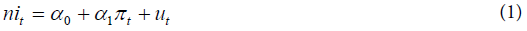Where, nit is the logarithm of the nominal interest rate; πt represents the inflation rate, and ut is the error term. The threshold ADL regression model of Fisher equationtakes the following form: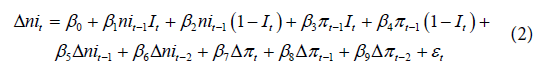Where, It denotes two indicators,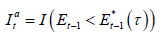and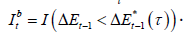In (2) if we adopt the first indicator, then we replace It with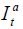and if the second indicator is adopted, then we replace It with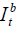.In either indicator, Et is the estimated error correction term. Note that the adjustment speed which is measured by βi (i = 1, 2, 3, 4) do vary in this set up which is what makes the threshold ADL model to be different than a standard ADL specification. Here, only two lags of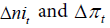are included in the regression so that we do not lose too many degrees of freedom. The lag-selection is guided by the partial autocorrelation function (PACF) of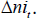Two tests are proposed for threshold cointegration by Li and Lee . One is due to Boswijk  denoted by BO test under which we test the coefficients of both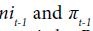in the testing regression. The second test known as BDM test is by Banerjee et al. . Under this test we add lead of πt-1 to the regression so that the asymptotic results are valid in the absence of strict exogeneity. The null hypotheses under both tests are outlined as: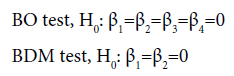In the absence of any rule for adopting Indicator A versus B in our model, the recommendation is to select the adjustment mechanism that is based on a set criterion such as the Akaike Information criteria (AIC) or Schwartz criteria (SC) .

#### Empirical Results and Policy Implications

Brazil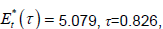, BO stat:26.169, BMD stat :22.463,AIC=1697.926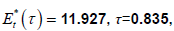, BO stat:48.034***, BMD stat :43.986***, AIC=2269.081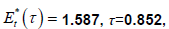, BO stat:22.547*, BMD stat:16.519*, AIC =697.51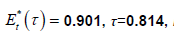, BO stat:17.388, BMD stat:16.827*, AIC =1008.491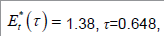, BO stat:12.599, BMD stat:11.742, AIC =888.655

Note:The critical values for BO statistic and BDM statistic are tabulated at Li and Lee's Table 1 of their paper.
The critical values of BO test for 10%, 5%, and 1% are 22.11, 24.67, and 30.09, respectively.
The critical values of BDM test for 10%, 5%, and 1% are 16.24, 18.66, and 23.72, respectively.
***, **, and * indicates significance at the 0.01, 0.05 and 0.1 levels, respectively.
The number in parenthesis indicates the robust t-statistic.

Table 3: Conditional threshold ADL model of fisher equation with indicator A.

Brazil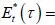-1.191,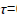0.153, BO stat :33.684***, BMD stat :31.905***, AIC =1691.0030.028,0.555, BO stat :44.095***, BMD stat :44.023***, AIC =2272.491-0.194,0.148, BO stat :17.524, BMD stat :11.699, AIC =702.326-0.029,0.496, BO stat :15.032, BMD stat :14.384, AIC =1010.7860.000,0.521, BO stat:15.86, BMD stat :13.053, AIC =885.452

Note:The critical values for BO statistic and BDM statistic are tabulated at Li and Lee's Table 1 of their paper. The critical values of BO test for 10%, 5%, and 1% are 20.90, 23.43, and 28.66, respectively.
The critical values of BDM test for 10%, 5%, and 1% are 14.53, 16.84, and 21.46, respectively.
***, **, and * indicates significance at the 0.01, 0.05 and 0.1 levels, respectively.
The number in parenthesis indicates the robust t-statistic.

Table 4: Conditional threshold ADL model of fisher equation with indicator B.

Our findings imply that if monetary policy results in 5% inflation in BRICS, the nominal interest rate in the economy of those 4 countries (Brazil, Russia, India, and China) would eventually also increase by 5%. Therefore, a change in the money supply shouldn’t have an effect on the real interest rate. If the real interest rate isn’t affected, then all changes in inflation must be reflected in the nominal interest rate, which is exactly what the Fisher effect claims.

#### Summary and Conclusion

The Fisher effect asserts that monetary policy that may result in x% inflation, eventually will push the nominal interest rate up by x%, leaving the real interest rate unchanged. We employ the ADL test for threshold cointegration recently introduced by Li et al.  to verify the Fisher effect in BRICS countries. The Monte Carlo simulations of Li et al.  shows that their test do not suffer from low power and have good size properties. Our empirical results support Fisher effect in BRICS countries, except in South Africa.

#### References

Citation: Bahmani-Oskooee M, Li JP, Chang T (2016) Revisiting Fisher Equation in BRICS Countries. J Glob Econ 4:210. Doi: 10.4172/2375-4389.1000210

Copyright: © 2016 Bahmani-Oskooee M, et al. This is an open-access article distributed under the terms of the Creative Commons Attribution License, which permits unrestricted use, distribution, and reproduction in any medium, provided the original author and source are credited.

Select your language of interest to view the total content in your interested language

Rome, Italy

Vienna, Austria

### 5th World Dermatology and Aesthetic Congress

Capetown, South Africa
##### Recommended Journals
Viewmore
###### Article Usage
• Total views: 8954
• [From(publication date): 9-2016 - Jan 21, 2020]
• Breakdown by view type
• HTML page views: 8827Can't read the image? click here to refresh